JMSLTM Numerical Library 7.2.0
com.imsl.math

## Class Spline2DLeastSquares

• All Implemented Interfaces:
Serializable, Cloneable

```public class Spline2DLeastSquares
extends Spline2D```
Computes a two-dimensional, tensor-product spline approximant using least squares.

The Spline2DLeastSquares class computes a tensor-product spline least-squares approximation to weighted tensor-product data. The input consists of data vectors to specify the tensor-product grid for the data, two vectors with the weights, the values of the surface on the grid, and the specification for the tensor-product spline. The grid is specified by the two vectors x = `xData` and y = `yData` of length
n = `xData.length` and m = `yData.length`, respectively. A two-dimensional array f = `fData` contains the data values which are to be fit. The two vectors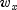= `xWeights` and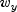= `yWeights` contain the weights for the weighted least-squares problem. The information for the approximating tensor-product spline can be provided using the `setXOrder`, `setYOrder`, `setXKnots` and `setYKnots` methods. This information is contained in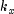= `xOrder`,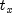= `xKnots`, and N = `xSplineSpaceDim` for the spline in the first variable, and in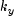= `yOrder`,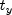= `yKnots` and M = `ySplineSpaceDim` for the spline in the second variable. This class computes coefficients for the tensor-product spline by solving the normal equations in tensor-product form as discussed in de Boor (1978, Chapter 17). The interested reader might also want to study the paper by Grosse (1980).

As the computation proceeds, we obtain coefficients c minimizing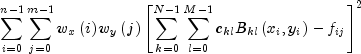where the function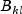is the tensor-product of two B-splines of orderand. Specifically, we have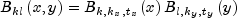The spline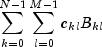and its partial derivatives can be evaluated using the `value` method.

Example 1, Serialized Form
• ### Constructor Summary

Constructors
Constructor and Description
```Spline2DLeastSquares(double[] xData, double[] yData, double[][] fData, int xSplineSpaceDim, int ySplineSpaceDim)```
Constructor for `Spline2DLeastSquares`.
• ### Method Summary

Methods
Modifier and Type Method and Description
`void` `compute()`
Computes a two-dimensional, tensor-product spline approximant using least squares.
`double` `getErrorSumOfSquares()`
Returns the weighted error sum of squares.
`int` `getXOrder()`
Returns the order of the spline in the x-direction.
`double[]` `getXWeights()`
Returns the weights for the least-squares fit in the x-direction.
`int` `getYOrder()`
Returns the order of the spline in the y-direction.
`double[]` `getYWeights()`
Returns the weights for the least-squares fit in the y-direction.
`void` `setXKnots(double[] xKnots)`
Sets the knot sequences of the spline in the x-direction.
`void` `setXOrder(int xOrder)`
Sets the order of the spline in the x-direction.
`void` `setXWeights(double[] xWeights)`
Sets the weights for the least-squares fit in the x-direction.
`void` `setYKnots(double[] yKnots)`
Sets the knot sequences of the spline in the y-direction.
`void` `setYOrder(int yOrder)`
Sets the order of the spline in the y-direction.
`void` `setYWeights(double[] yWeights)`
Sets the weights for the least-squares fit in the y-direction.
• ### Methods inherited from class com.imsl.math.Spline2D

`derivative, derivative, getCoefficients, getXKnots, getYKnots, integral, value, value`
• ### Methods inherited from class java.lang.Object

`clone, equals, finalize, getClass, hashCode, notify, notifyAll, toString, wait, wait, wait`
• ### Constructor Detail

• #### Spline2DLeastSquares

```public Spline2DLeastSquares(double[] xData,
double[] yData,
double[][] fData,
int xSplineSpaceDim,
int ySplineSpaceDim)```
Constructor for `Spline2DLeastSquares`.
Parameters:
`xData` - a `double` array containing the data points in the x-direction.
`yData` - a `double` array containing the data points in the y-direction.
`fData` - a `double` matrix of size `xData.length` by `yData.length` containing the values to be approximated.
`xSplineSpaceDim` - an `int` scalar value specifying the linear dimension of the spline subspace for the x variable. It should be smaller than `xData.length` and greater than or equal to `xOrder` (whose default value is 4).
`ySplineSpaceDim` - an `int` scalar value specifying the linear dimension of the spline subspace for the y variable. It should be smaller than `yData.length` and greater than or equal to `yOrder` (whose default value is 4).
• ### Method Detail

• #### compute

`public void compute()`
Computes a two-dimensional, tensor-product spline approximant using least squares.
• #### getErrorSumOfSquares

`public double getErrorSumOfSquares()`
Returns the weighted error sum of squares.
Returns:
a `double` scalar containing the weighted error sum of squares.
• #### getXOrder

`public int getXOrder()`
Returns the order of the spline in the x-direction.
Returns:
an `int` scalar containing the order of the spline in the x-direction.
• #### getXWeights

`public double[] getXWeights()`
Returns the weights for the least-squares fit in the x-direction.
Returns:
a `double` array containing the weights for the least-squares fit in the x-direction.
• #### getYOrder

`public int getYOrder()`
Returns the order of the spline in the y-direction.
Returns:
an `int` scalar containing the order of the spline in the y-direction.
• #### getYWeights

`public double[] getYWeights()`
Returns the weights for the least-squares fit in the y-direction.
Returns:
a `double` array containing the weights for the least-squares fit in the y-direction.
• #### setXKnots

`public void setXKnots(double[] xKnots)`
Sets the knot sequences of the spline in the x-direction.
Parameters:
`xKnots` - a `double` array of size `xSplineSpaceDim + xOrder` specifying the knot sequences of the spline in the x-direction. Default knot sequences are selected by the class.
• #### setXOrder

`public void setXOrder(int xOrder)`
Sets the order of the spline in the x-direction.
Parameters:
`xOrder` - an `int` scalar value specifying the order of the spline in the x-direction. `xOrder` must be at least 1. Default: `xOrder` = 4, implying a tensor-product cubic spline.
• #### setXWeights

`public void setXWeights(double[] xWeights)`
Sets the weights for the least-squares fit in the x-direction.
Parameters:
`xWeights` - a `double` array of size `xData.length` specifying the weights for the least-squares fit in the x-direction. Default: all weights are equal to 1.
• #### setYKnots

`public void setYKnots(double[] yKnots)`
Sets the knot sequences of the spline in the y-direction.
Parameters:
`yKnots` - a `double` array of size `ySplineSpaceDim + yOrder` specifying the knot sequences of the spline in the y-direction. Default knot sequences are selected by the class.
• #### setYOrder

`public void setYOrder(int yOrder)`
Sets the order of the spline in the y-direction.
Parameters:
`yOrder` - an `int` scalar value specifying the order of the spline in the y-direction. `yOrder` must be at least 1. Default: `yOrder` = 4, implying a tensor-product cubic spline.
• #### setYWeights

`public void setYWeights(double[] yWeights)`
Sets the weights for the least-squares fit in the y-direction.
Parameters:
`yWeights` - a `double` array of size `yData.length` specifying the weights for the least-squares fit in the y-direction. Default: all weights are equal to 1.
JMSLTM Numerical Library 7.2.0